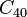# Cyclic group:Z40

View a complete list of particular groups (this is a very huge list!)[SHOW MORE]

## Definition

This group, denoted$C_{40}$,$\mathbb{Z}_{40}$, or$\mathbb{Z}/40\mathbb{Z}$, is defined in the following equivalent ways:

• It is the unique (up to isomorphism) cyclic group of order 40, i.e., it is a group of integers modulo n where$n = 40$.
• It is the external direct product of cyclic group:Z8 and cyclic group:Z5, i.e., is it$\mathbb{Z}_8 \times \mathbb{Z}_5$.

## GAP implementation

### Group ID

This finite group has order 40 and has ID 2 among the groups of order 40 in GAP's SmallGroup library. For context, there are groups of order 40. It can thus be defined using GAP's SmallGroup function as:

SmallGroup(40,2)

For instance, we can use the following assignment in GAP to create the group and name it$G$:

gap> G := SmallGroup(40,2);

Conversely, to check whether a given group$G$ is in fact the group we want, we can use GAP's IdGroup function:

IdGroup(G) = [40,2]

or just do:

IdGroup(G)

to have GAP output the group ID, that we can then compare to what we want.

### Other descriptions

Description Functions used
CyclicGroup(40) CyclicGroup
DirectProduct(CyclicGroup(8),CyclicGroup(5)) CyclicGroup, DirectProduct